Fill Table with Last Survey Result - Excelerator BI

# Fill Table with Last Survey Result

I have come across this problem a few times recently. There was no immediately obvious solution that worked at first. I knew what I wanted to do but I couldn’t get it to work – but now I have a solution and I want to share it for the benefit of others.

## First, let me explain what I mean by “Last Survey Result”

The scenario I am talking about here is one where there is an event that occurs infrequently in time – say once every couple of months, and you want to refer to the value of the last event in your DAX formulae until there is a new event to replace it. So one example is an “Audit” or “Survey” which was a case with Satoshi (participant at my recent Brisbane training course) or it could also be the “last handicap the golfer had” which was the problem that Michael had (participant at my last Sydney Power Pivot course). In both of these scenarios, we want to be able to refer to the “last value” of the event (survey or golf handicap) and keep referring to that value from the last recorded event until that value is replaced with a new value at some point in the future. So time marches on, but I still want to see that last value in my pivot tables for the current date. This is also referred to as “events in progress” by The Italians.

## Why is this such a hard problem?

Before I answer this, let’s talk about a scenario that IS NOT a problem. Let’s take a simple Adventure Works data model. In this case you have a number of lookup tables sitting up top, and each lookup table is connected to the data table below (in this case the data table is sales)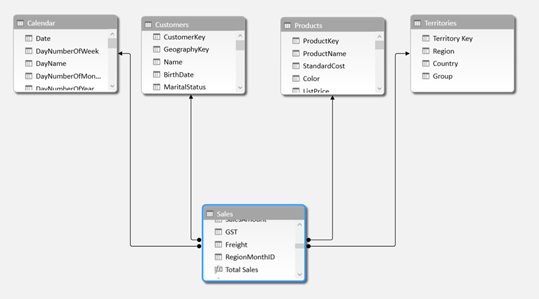When you put a column from your lookup table into a pivot table (eg Months on rows, and Years on columns), the filter context from the pivot table propagates through the relationship and your measure (say Total Sales) will only return the sales that occurred in that Month/Year. So you might end up with a pivot table like this.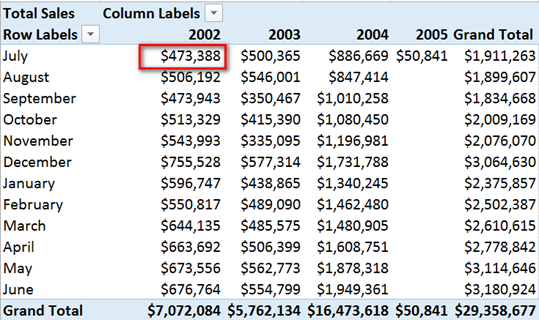All the sales that relate to Year = 2002, Month = July, will end up in that first cell – and so on.

In the case of our “last survey result” scenario, you may get a survey result in Jan, and then don’t have another survey until March as shown in this sample data below.

### Sample Test Data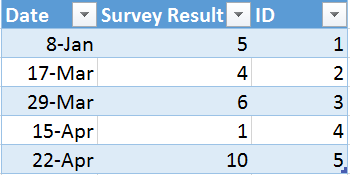If I then put Calendar[Date] or Calendar[Months] on Rows, the initial filter context from your Calendar table will “filter out” every date other than the dates of the actual surveys. So what I want to see in my pivot is very different to what I actually get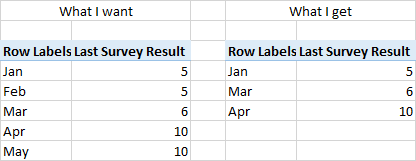## So how to solve this problem?

Well as so often is the case when I get stuck, I start Googling for solutions, and if that doesn’t work then I “phone a friend” for advice. And that cry for help normally goes out to Scott Senkeresty from http://tinylizard.com. You see one of the things I have learnt about DAX over the last 12 months is that experience is everything. So a quick shout of “help” across the Pacific, and Scott shot me back a link (that Scott remembered reading) on SQLBLOG.COMwhere Alberto Ferrari described a similar problem to mine. With my relevant reference material in hand, I set about solving my problem taking the learnings that Alberto provided in this post.

### First delete the relationship

The first thing I realised after starting to read Alberto’s post was that I needed to delete the relationship between the calendar table and the data table. As I described above, when you REALLY WANT to limit the sales records to “just those that occurred this month” then you REALLY DO want that relationship in place. But when you are trying to fill a table of dates with the “last valid entry” even if it didn’t occur on that actual date, then the table connection is you enemy. So the first thing to do is get rid of that relationship.

### Then I wrote some interim measures

If there is one thing I would like written on my grave stone, I would like it to say “Nice guy, wrote a lot of interim measures”. Interim measures are like the steps on a ladder. Take one step at a time and you can scale great heights. Try to take one gigantic step all at once an you are probably going to get into trouble.

So I set about my first interim measure to find which of the last surveys (or handicap etc) is the last one in the filter context. Well one really valuable trick to find the “last value” in a table is to add an ID column, like you can see in my sample data earlier in this post. Now a word of caution here. DAX is a Columnar Data Store and it has excellent compression algorithms. The number one enemy of compression in DAX is UNIQUE VALUES. An ID column by definition is a column of unique values. So be very careful when using such a column on a data table. In my case, the “survey” occurs relatively infrequently, so there are not many rows in the table, and hence the ID column is not really an issue. But avoid putting an ID column on a 50 million row data table unless you really need it!

So the first measure I wrote was one that found the last Survey ID for the current date. Put Calendar[Date] on Rows, and then I wrote the following measure.

```Last Survey ID:=CALCULATE(max(Survey[ID]),
FILTER ( Survey,
Survey[Date] <=LASTDATE(Calendar[Date])
)
)```

This measure uses the formula MAX ( ) to extract the highest value of Survey[ID] from my table in the current filter context. The highest value of Survey[ID] is always the last survey, and the FILTER( ) function is ensuring that we first INSPECT the pivot table to find out what the LASTDATE in the current filter context is before returning the last Survey[ID] for that filter context..

So as you can see in the Pivot Table below, this measure returns “1” for the last ID for the dates 8 Jan – 16 Jan and then switches to “2” from 17 Jan – and so on. Note that LASTDATE ( ) respects the initial filter context from the pivot table, so rather than returning the “last date” in my entire calendar table, it returns the “last date” from my filter context.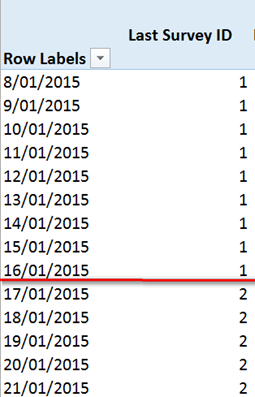## OK great, now what?

So now I know that I want to extract survey ID 1 between 8 Jan and 16 Jan, and I want to use Survey ID 2 from 17 Jan etc (see the workbook for the full set of data). So then I wrote this measure to extract the “last survey result” based on the Survey ID that I knew was the right survey

```Last Survey Result Does not work:=
CALCULATE (
MAX ( Survey[Survey Result] ),
FILTER ( Survey ,
Survey[ID] = [Last Survey ID]    )
)
```

Now let’s take a look at this measure above. Firstly I have a calculate to modify the initial filter context. I want to calculate the value of “MAX(Survey[Survey Result]” but only after filtering the rows of my survey table so that ONLY 1 SINGLE ROW REMAINS. The only row I am interested in in this case is the row where survey[ID] = [Last Survey ID]. As you can see in my image above, I have correctly identified WHICH survey ID I need to use, and so logic tells me that I just need to filter my survey table before harvesting the MAX( ) of the result, and I am done! But alas, it didn’t work.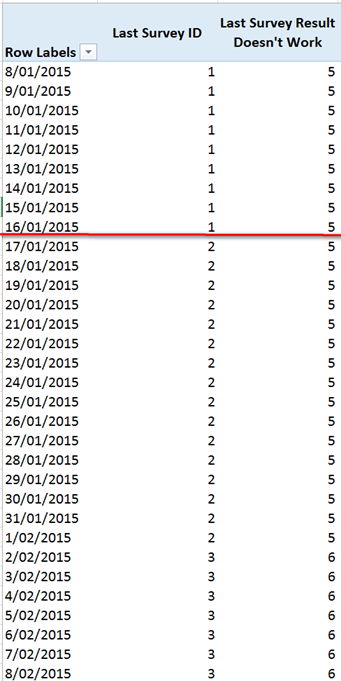As you can see in the above Pivot Table, the value of “Last Survey Doesn’t Work” does not correctly change from the value 5 to 4 on 17 Jan. Now this really troubled me A LOT. WHY DIDN’T IT WORK!!! Now before I answer that question, let me spend a moment talking about the importance of quality relevant test data. If you look at my sample test data above, you will note that my sample survey for Jan 8 had a result of 5, and then my sample survey result for 17 Jan had a result of 4. I did this very deliberately. I was going to use MAX ( ) as the aggregation function to extract the “one and only one” surviving value from my FILTER( ) function. By deliberately using a smaller survey result value here, I was able to put in a “trap” to catch possible problems. Always try to design your test data to help you trap potential errors.

So on 17 Jan, I was expecting the survey result to be 4, but I got 5. Why, why, why?! Now I was getting annoyed. I could see the value of LAST SURVEY ID in my pivot, and I was passing that value to my filter function (via the measure). This SHOULD filter the survey table so only this single ID would survive the FILTER. But it wasn’t working.

## Back to the blog on sqlblog.com

So I read through the blog post that Scott sent me (written by Alberto Ferrari). What I noticed in his blog post was that Alberto didn’t use any interim measures (like my [Last Survey ID’] measure.) I started to think about nested Filter formulae and it occurred to me that maybe, just maybe this was the problem. So on a hunch, I copied the DAX from my measure [Last Survey ID] and pasted into the secondary measure [last survey result doesn’t work] and hence ended up with this.

```Last Survey Result:=CALCULATE (
MAX ( Survey[Survey Result] ),
FILTER (Survey ,
Survey[ID]
= CALCULATE (
LASTNONBLANK ( Survey[ID], 1 ),
FILTER (
Survey,
Survey[Date] <= LASTDATE ( Calendar[Date] )
)
)
)
)```

So now we have a scenario where I am filtering the Survey table where Survey[ID] is equal to another calculate formula that goes ahead and calculates the related survey ID “on the fly” during the formula evaluation. Now I have to be honest here. I don’t really know why this works this way and not the other way – I would need to ask Alberto or Marco to explain that.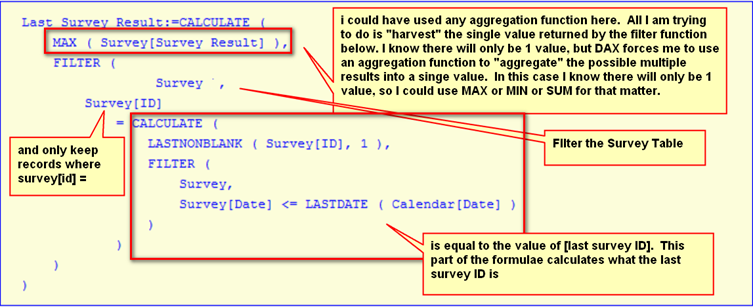Here is the sample Workbook if you want to download it and check it out.

### 3 thoughts on “Fill Table with Last Survey Result”

1.Hi Matt,

Thank you very much for sharing this on the blog.

I am a newbie to the PowerPivot world but will come back to the blog to develop my undestanding in DAX formulas and the DAX engine.

This post is easy to read and well written to highlight the problem and suggest a solution step by step.

Kind regards,

Sato

1.Thanks for the links Chris. One of the challenges with finding solutions to such problems is knowing what to search for. I will definitely look into these links.

Matt

Scroll to Top